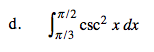### Home > CALC > Chapter 7 > Lesson 7.4.3 > Problem7-199

7-199.
1. Examine the integrals below. Consider the multiple tools available for integrating and use the best strategy. After evaluating each integral, write a short description of your method. Homework Help ✎

1.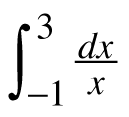2.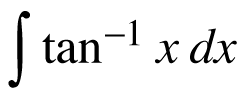3.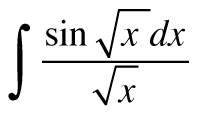4.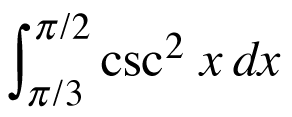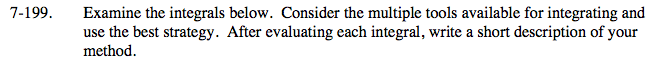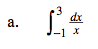Rewrite properly (with limits) before evaluating.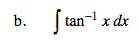Use integration by parts after you rewrite the integrand as arctan(x).
Let f(x) = arctan(x) and dg = dx.
Then df = _______ and g(x) = _______.
Warning: You will have to use U-substitution to evaluate the integral.

$\text{Use }U\text{-Substitution}. \text{ \ \ Let }U=\sqrt{x}.$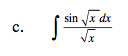$\text{Recall that }\frac{d}{dx}\text{cot}x=-\text{csc}^{2}x.$